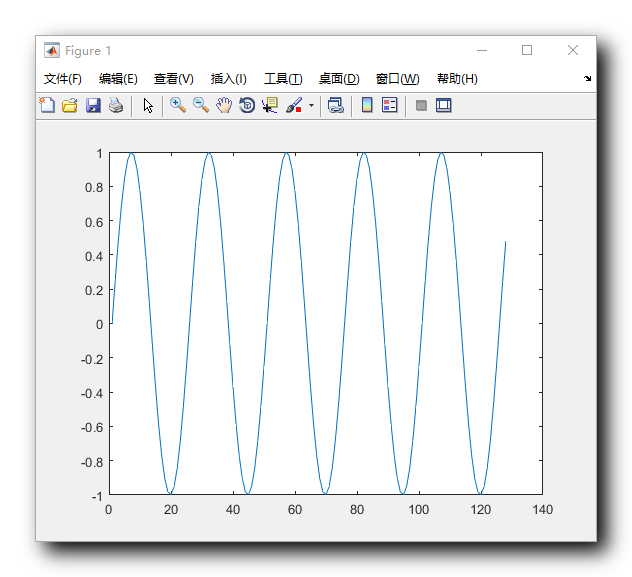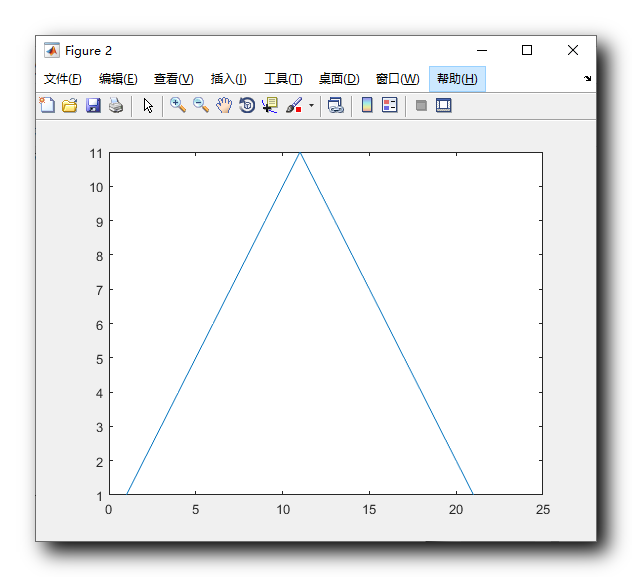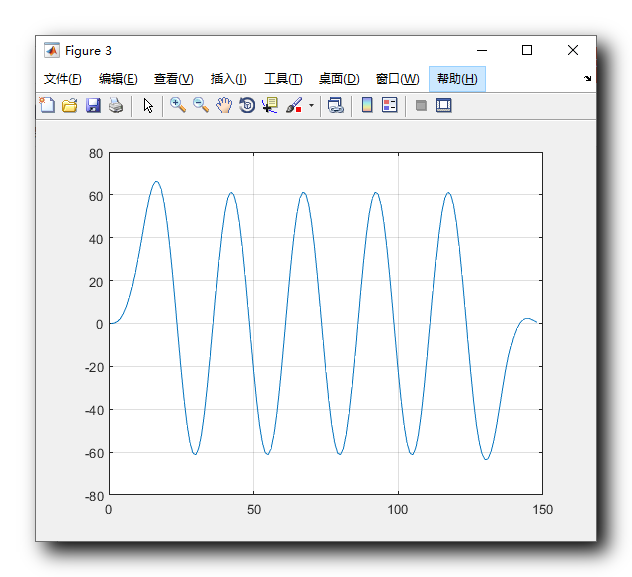• 实验四-使用matlab实现卷积的运算
2021-05-08 14:15:19

一 实验目的

1、 学习MATLAB 语言的编程方法及熟悉MATLAB 指令；

2、

深刻理解卷积运算，利用离散卷积实现连续卷积运算；

二 实验内容

1、 完成)(1t f 与)(2t f 两函数的卷积运算 其中：)4()()(),

()(221--==-t u t u t f t u e

t f t

在一个图形窗口中，画出)(1t f 、)(2t f 以

及卷积结果。要求每个坐标系有标题、坐标轴名称。

p = ; %定义时间间隔 t= 0:p:10;

%定义时间向量

f1=exp(-2*t).*u(t); %将f (t )表示出来 f2=u(t)-u(t-4); f=conv(f1,f2); subplot(1,2,1);

plot(t,f1,t,f2); title('f1=e^-2t*u(t)'' / ''f2=u(t)-u(t-4)');

xlabel('t(sec)'); % 这行代码是给出x 坐标的标签 ylabel('f(t)'); grid on ；

subplot(1,2,2);

plot(f); title('f=f1*f2');

xlabel('t(sec)'); % 这行代码是给出x 坐标的标签 ylabel('f')

grid on

更多相关内容
• matlab实现的（2,1,3）卷积码编译码程序，译码使用维特比译码，欢迎大家下载
• 利用MATLAB卷积工具处理机械加工信号技术探析.pdf
• matlab循环卷积函数,使用矩阵实现，用于理解循环卷积。分2部分，一部分为循环移位，第二部分给矩阵赋值、计算循环卷积
• 多种方法进行圆周卷积MATLAB实现（使用定义、DFT、和卷积计算）
• 卷积滤波器matlab代码使用MATLAB进行信号处理的各种代码 噪音 均线 相关性 上采样 下采样 Z变换 卷积 频率相位组延迟：全通滤波器 低通滤波器：FIR，巴特沃斯，切比雪夫，贝塞尔，椭圆形
• 一、Matlab 卷积和多项式乘法 conv 函数、 二、使用 matlab 代码求卷积并绘图

# 一、Matlab 卷积和多项式乘法 conv 函数

Matlab 文档地址 : https://ww2.mathworks.cn/help/matlab/ref/conv.html

函数语法 :

// 返回向量 u 和 v 的卷积。如果 u 和 v 是多项式系数的向量，对其卷积与将这两个多项式相乘等效。
w = conv(u,v)
// 返回如 shape 指定的卷积的分段。
// 例如，conv(u,v,'same') 仅返回与 u 等大小的卷积的中心部分，
// 而 conv(u,v,'valid') 仅返回计算的没有补零边缘的卷积部分。
w = conv(u,v,shape)


u u 是 " 输入序列 " , v v 是 " 单位脉冲响应 " ;

# 二、使用 matlab 代码求卷积并绘图

求下面的 " 线性时不变系统 " 的 输出序列 ;

输入序列 : x ( n ) = s i n ( 2 π f 0 n / F s ) ,   0 ≤ n ≤ 127 x(n) = sin(2\pi f_0n/F_s) , \ 0 \leq n \leq 127 ,

f 0 = 0.4 k H z , F s = 10 k H z f_0 = 0.4kHz , F_s = 10kHz

单位脉冲响应 :

h ( n ) = { 1 , 2 , 3 , 4 , 5 , 6 , 7 , 8 , 9 , 10 , 11 , 10 , 9 , 8 , 7 , 6 , 5 , 4 , 3 , 2 , 1 } h(n) = \{ 1,2,3,4,5,6,7,8,9,10,11,10,9,8,7,6,5,4,3,2,1 \}

上述系统的输出就是 " 输入序列 " 与 " 输出序列 " 的卷积 ;

f 0 = 0.4 k H z f_0 = 0.4kHz 表示模拟信号频率是 0.4 k H z 0.4kHz ,

F s = 10 k H z F_s = 10kHz 表示数字采样频率是每秒采集 10 k 10k 个样本 , 也就是 10000 10000 个样本 ;

x ( n ) x(n) 默认是一个无限序列 , 为了方便计算 , 这里为其加了一个区间 0 ≤ n ≤ 127 0 \leq n \leq 127 ;

数字频率计算 :

数字频率 ( 单位 Hz ) : f = f 0 / F s = 0.4 / 10 = 0.04 f = f_0 / F_s = 0.4 / 10 = 0.04

matlab 代码示例 :

%清除内存
clear;

%输入序列 , 模拟信号频率 0.4kHz , 采样频率 10kHz
% n 的取值范围是 0 到 127 闭区间整数序列
x=sin(2 * pi * 0.4 * (0:127) / 10);

%单位脉冲响应
%1,2,3,4,5,6,7,8,9,10,11,10,9,8,7,6,5,4,3,2,1
%1 ~ 10 索引的序列就是 1,2,3,4,5,6,7,8,9,10
h(1:10)=(1:10);
%11 索引的序列是 11
h(11)=11;
%12 ~ 21 索引的序列是 10,9,8,7,6,5,4,3,2,1
h(12:21)=(10:-1:1);

%求 " 单位脉冲响应 " 序列的频谱
hf = freqz(h, 1, 1024);
%求 " 输入序列 " 的频谱
xf = freqz(x, 1, 1024);

%求卷积 , 线性时不变系统的输出序列就是 输入序列 与 单位脉冲响应 之间的卷积
y=conv(h,x);

%建立幕布
figure;
%绘制 "输入序列" 图像
plot(x);

%建立幕布
figure;
%绘制 "单位脉冲响应" 图像
plot(h);

%建立幕布
figure;
%绘制 y 函数的值 , 也就是卷积计算结果
plot(y);

% 打开网格
grid on;


输入序列 x ( n ) x(n) 的函数图像如下 :单位脉冲响应 h ( n ) h(n) 函数图像如下 :输出序列 y ( n ) y(n) 图像如下 :展开全文数字信号处理
• 本代码是基于Matlab的卷积神经网络源代码案例，本代码本人使用Matlab2014b可以正常运行。欢迎大家留言评论，互相学习研讨。
• 用卷积滤波器matlab代码Matlab卷积自动编码器 卷积自动编码器的成本函数（cautoCost2.m）和成本梯度函数（dcautoCost2.m）。 网络体系结构相当有限，但是这些功能对于将输入与一组过滤器进行卷积然后重构的无监督...
• MATLAB实现卷积神经网络，并对图像进行特征提取 文件列表： CNN CNN\cnnapplygrads.m CNN\cnnbp.m CNN\cnnff.m CNN\cnnnumgradcheck.m CNN\cnnsetup.m CNN\cnntest.m CNN\cnntrain.m CNN\expand.m CNN\...
• 卷积滤波器matlab代码这是什么？ S ignal P rocessing O RG N OTES 这是使用组织模式组织小型软件项目的示例。 这里的项目（主要是C ++），包括几个相关的内容： 一个（模板）类： Cb 以规范形式（又称直接形式II...
• 卷积滤波器matlab代码VDID Jae Woong Soh和Nam Ik Cho [] 环境环境 Ubuntu 18.04 （> = 1.8） CUDA 11.2和cuDNN Python 3.7 抽象的 卷积神经网络（CNN）在大规模数据集的帮助下在图像去噪方面表现出出色的性能。 ...
• 计算卷积matlab代码 Computer-Version-Notes 计算机视觉入门 常用库 OpenCV Open Source Computer Vision Library，由一系列 C 函数和少量 C++ 类构成，同时提供了Python、Ruby、MATLAB等语言的接口，实现了图像...
• 利用MATLAB 求解卷积的方法分类 求法一：求有序列A (n )=1 n=3,4,5,6,7,8,9,,10,11,1,2.B(n)=1 n=2,3,4,5,6,7,8,9,. 代码：n1=3;n2=12;>> m1=2;m2=9;>> a=ones(1,(n2-n1+1));>> b=ones(1,(m2-m1+1...

利用MATLAB 求解卷积的方法分类 求法一：

求有序列A (n )=1 n=3,4,5,6,7,8,9,,10,11,1,2.B(n)=1 n=2,3,4,5,6,7,8,9,. 代码：

n1=3;n2=12;

>> m1=2;m2=9;

>> a=ones(1,(n2-n1+1));

>> b=ones(1,(m2-m1+1));

>> c=conv(a,b);

>> nc1=n1+m1;nc2+m2+n2;

nc1=n1+m1;nc2=m2+n2;

>> kc=nc1:nc2;

>> kc,c

stem(kc,c),text(18,6,'非平凡法')

>> CC=[zeros(1,kc(1)),c];

kc=0:(n2+m2);

>> stem(kc,CC),text(18,6,'非平凡法')

求法二：

N1=3;N2=12;

a=ones(1,N2+1);a(1:N1)=0;

M1=2;M2=9;

b=ones(1,M2+1);b(1:M1)=0;

c=conv(a,b);

kc=0:(N2+M2);

kc,c

subplot(2,1,1),stem(kc,c),text(20,6,'0 起点法')

求法三：

求有限序列与无限序列的卷积

clear

>> M=4;

>> h=[0.1,0.35,-0.42,-0.05,0.15];

>> N=10000;

>>rng default %恢复matlab 启动时默认的全局随机流。

>> u=randsrc(1,N);

>>tic

>> y_filter=filter(h,1,u);

>> t_filter=toc;

>> tic

>> ct=[u(1),zeros(1,M)];

>> ut=toeplitz(ct,u);

>> y_toe=h*ut;

>> t_toe=toc;

>> %卷积指令法

>> tic

>> y_conv=conv(h,u);

>> t_conv=toc;

>> y_conv(N+1:end)=[];

filter 是一维数字滤波器

使用方法:

Y = filter(B,A,X) ，输入X 为滤波前序列，Y 为滤波结果序列，B/A 提供滤波器系数，B 为分子， A 为分母

整个滤波过程是通过下面差分方程实现的:

a(1)*y(n) = b(1)*x(n) + b(2)*x(n-1) + ... + b(nb+1)*x(n-nb) - a(2)*y(n-1) - ... - a(na+1)*y(n-na)

[Y,Zf] = filter(B,A,X,Zi)，输入X 为滤波前序列，Y 为滤波结果序列，B/A 提供滤波器系数，B 为分子， A 为分母，

在MATLAB 中，可以用函数y=filter(p,d,x)实现差分方程的仿真，也可以用函数 y=conv(x,h)计算卷积，用y=impz(p,d,N)求系统的冲激响应。

实现差分方程

先从简单的说起：

filter([1,2],1,[1,2,3,4,5])

实现 y[k]=x[k]+2*x[k-1]

y=x+2*0=1%(x之前状态都用0)

y=x+2*x=2+2*1=4

a. 下面程序是用来实现h 和x 的卷积得，分别用了filter 和conv 函数，两者函数得出的结果一样。

h = [3 2 1 -2 1 0 -4 0 3]; % impulse response

x = [1 -2 3 -4 3 2 1]; % input sequence

y = conv(h,x);

n = 0:14;

subplot(2,1,1);

stem(n,y);

xlabel('Time index n'); ylabel('Amplitude');

title('Output Obtained by Convolution'); grid;

x1 = [x zeros(1,8)];

y1 = filter(h,1,x1);

subplot(2,1,2);

stem(n,y1);

xlabel('Time index n'); ylabel('Amplitude');

title('Output Generated by Filtering'); grid;

要实现下式的冲击响应和阶跃响应，可以分别采用三种方法。

y[n]+0.75y[n-1]+0.125y[n-2]=x[n]-x[n-1]。

b. 单位冲激响应：

(1)用filter 函数

a1=[1,0.75,0.125];

b1=[1,-1];

n=0:20;

x1=[1 zeros(1,20)];

y1filter=filter(b1,a1,x1);

stem(n,y1filter);

title('y1filter');

xlabel('x');

ylabel('y');

(2)用conv 函数

a1=[1,0.75,0.125];

b1=[1,-1];

x1=[1 zeros(1,10)];

[h]=impz(b1,a1,10);

y1conv=conv(h,x1);

n=0:19;

stem(n,y1conv,'filled')

(3)用impz 函数

a1=[1,0.75,0.125];

b1=[1,-1];

impz(b1,a1,21);

c. 单位阶跃响应：

(1)用filter 函数

a1=[1,0.75,0.125];

b1=[1,-1];

n=0:20;

x2=ones(1,21);

y1filter=filter(b1,a1,x2);

stem(n,y1filter);

title('y1filter_step');

xlabel('x');

ylabel('y');

(2)用conv 函数

a1=[1,0.75,0.125];

b1=[1,-1];

x2=ones(1,21);

[h]=impz(b1,a1,20);

y1=conv(h,x2);

y1conv=y1(1:21); %为何y1conv 要取y1中1：21的值，解释见

n1=0:20; %y2à单位阶跃响应à用conv 函数中注释

stem(n1,y1conv,'filled');

title('y1conv');

xlabel('n');

ylabel('y1[n]');

(3)用impz 函数

a=[1,0.75,0.125];

b=1;

impz(b,a)

即y=filter(p,d,x)用来实现差分方程，d 表示差分方程输出y 的系数，p 表示输入x 的系数，而x 表示输入序列。输出结果长度数等于x 的长度。

而y=conv(x,h)是用来实现卷级的，对x 序列和h 序列进行卷积，输出的结果个数等于x 的长度与h 的长度之和减去1。

y=impz(p,d,N)是用来实现冲击响应的，d 和p 的定义见filter ，N 表示冲击响应输出的序列个数。

展开全文• MATLAB卷积神经网络案例，适合初学者使用，需要安装深度学习工具箱
• matlab卷积实验报告matlab卷积实验报告篇一：MATLAB实验报告卷积　实 验 报 告　学院： 机电 班级： 姓名： 学号：　实验名称：连续时间信号卷积运算的MATLAB实现　1. 实验目的：掌握卷积的概念及计算方法　2. 熟悉...

matlab卷积实验报告

matlab卷积实验报告

篇一：MATLAB实验报告卷积　　实 验 报 告　　学院： 机电 班级： 姓名： 学号：　　实验名称：连续时间信号卷积运算的MATLAB实现　　1. 实验目的：掌握卷积的概念及计算方法　　2. 熟悉通过调用conv()函数求解连续时间信号卷积的数值分析法　　实验环境：MATLAB 6.5.1软件　　实验要求：　　1、已知信号f1(t)=t/2*[ε(t)- ε(t-2)], f2(t)= [ε　　(t)- ε(t-1)]，通过调用conv()函数编程实现卷积计算y(t)= f1(t)* f2(t),画出波形。　　2、已知信号f(t)=e-t *ε(t), h(t)= t2 *e-2t *ε(t),y(t)= f(t)* h(t)　　(1)用符号分析法编程实现计算y(t)的理论解；　　(2)过调用conv()函数编程实现卷积计算y(t)的数值解并画图　　实验程序及结果：　　第一题：　　M文件　　(1)function f=uCT(t)　　f=(t>=0);　　主程序：　　k1=0:p:2;　　k2=0:p:1;　　f1=k1/2.*[uCT(k1)-uCT(k1-2)];　　f2=uCT(k2)-uCT(k2-1);　　y=conv(f1,f2)*p;　　k0=k1(1)+k2(1);　　k3=length(f1)+length(f2)-2;　　k=k0:p:k3*p+k0;　　subplot(311)　　plot(k1,f1);　　xlabel('t')　　ylabel('f1(t)')　　axis([-0.5 2.5 -0.5 1.5])　　grid on　　subplot(312);　　plot(k2,f2)　　grid on　　axis([-0.5 2.5 -0.5 1.5])　　xlabel('t')　　ylabel('f2(t)')　　subplot(313)　　axis([-0.5 4 -0.5 1.5])　　grid on　　xlabel('t')　　ylabel('f1(t)*f2(t)')　　实验结果： 　　第二题： 　　M文件　　function f=uCT(t)　　f=(t>=0);　　主程序：　　syms tao　　>> t=sym('t','positive');　　>> f=exp(-t);　　>> h=t^2*exp(-2*t);　　>> fh_tao=subs(f,t,tao)*subs(h,t,t-tao); >> yt=int(fh_tao,0,t) 　　yt = 　　-(-2*exp(t)+t^2+2*t+2)/exp(t)^2 　　p=0.01;　　k1=0:p:3;　　k2=0:p:12;　　f=exp(-k1).*uCT(k1);　　h=(k2).^2.*exp(-2.*k2).*uCT(k2); y=conv(f,h)*p;　　k0=k1(1)+k2(1);　　k3=length(f)+length(h)-2;　　k=k0:p:k3*p+k0;　　subplot(221)　　plot(k1,f);　　xlabel('t')　　ylabel('f(t)')　　grid on　　axis([-0.5 3.5 -0.5 1.5])　　subplot(222)　　plot(k2,h);　　xlabel('t')　　ylabel('h(t)')　　grid on　　axis([-0.5 11 -0.05 0.2])　　subplot(223)　　plot(k,y);　　grid on　　xlabel('t')　　ylabel('f(t)*h(t)数值')　　axis([-0.5 15 -0.01 0.1])　　subplot(224)　　yt =-(-2.*exp(k)+k.^2+2.*k+2)./exp(k).^2; plot(k,yt);　　grid on　　xlabel('t')篇二：实验五 使用matlab实现卷积的运算　　实验五 使用matlab实现卷积的运算　　一 实验目的　　1、 2、　　二 实验内容　　学习MATLAB语言的编程方法及熟悉MATLAB指令； 深刻理解卷积运算，利用离散卷积实现连续卷积运算；　　1、 完成f1(t)与f2(t)两函数的卷积运算 其中：f1(t)?e?2tu(t),　　f2(t)?u(t)?u(t?4)在一个图形窗口中，画出f1(t)、　　f2(t)以及卷积结果。要求每个坐标系有标题、坐标轴名称。 >> p=0.1;

展开全文• 实验五 使用matlab实现卷积的运算一 实验目的学习MATLAB语言的编程方法及熟悉MATLAB指令；深刻理解卷积运算，利用离散卷积实现连续卷积运算；二 实验内容完成与两函数的卷积运算其中：在一个图形窗口中，画出、以及...
• 卷积神经网络Matlab实现，使用matlab2021a，生成两类幅值不同的随机序列,作为两类待分类样本。可训练，可识别。
• 312卷积码编码与213卷积码编码，各自的matlab代码。卷积码编码程序使用状态机思路来编码，文件夹里除了两个.m文件，也包含了它们的状态图与网格图。
• 相比BP、GRNN、RBF、NARX神经网络的简单结构，深度神经网络结构更加复杂，比如卷积神经网络CNN，长短时序神经网络LSTM等，matlab集成了深度学习工具箱，可输入如下指令调用： Deep Network Designer ...dnn 深度学习
• 这是一个可用于卷积两个离散信号（或矩阵）的函数。 它不使用内置的 'conv' 函数。 与 'conv' 函数的语法相同。 事实上，这是对 'conv' 函数的替代。
• 摘 要：介绍了用MATLAB软件计算连续时间信号卷积的... 两个离散时间信号相卷积，可直接利用MATLABM文件中的conv函数；两个连续时间信号相卷积若采用数值解，首先要对连续时间信号采样得到离散信号f1和f2，再用con连续时间信号 开发板
• 要在Matlab使用现成的3D ConvNet，需要一个高级包装，例如in或vl_simplenn.m 。 安装 一步步： 在根目录中运行make_all.m以创建mex文件如果启用GPU，则需要CUDA工具包 在根目录中运行setup_path.m以添加路径 （可...
• 卷积定理 一实验目的 通过本实验验证卷积定理掌握利用 DFT 和 FFT 计算线性卷积的方法 二 实验原理 时域圆周卷积在频域上相当于两序列 DFT 的相乘因而可以采用 FFT 的 算法来计算圆周卷积当满足 L ?N1 ?N 2 ?1时线性...
• 使用MATLAB中的内核进行图像卷积。 使用以下代码运行： 图片= im2double（imread（'cameraman.tif'））; kernelA =个（5）/ 25; extendedA = extended_convolution（image，kernelA）; 图（'名称'，'扩展卷积'）; ...
•图像处理
• matlab卷积神经网络去噪代码 1. 项目介绍 1.1 项目的背景 该项目是为了研究基于深度卷积神经网络的图像去噪算法，是利用DnCNN模型，但是为了比较该算法的效果，另外实现了四种传统的图像去噪算法（均值滤波、中值...
• matlab卷积运算程序，不利用conv进行计算...

# 利用matlab卷积matlab 订阅